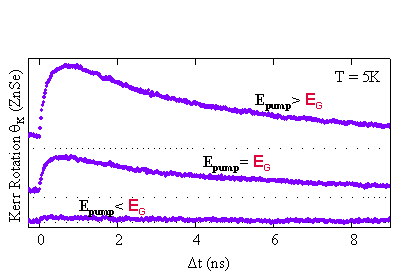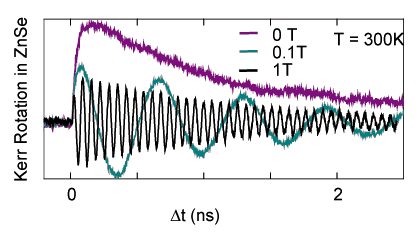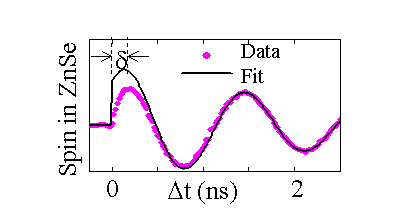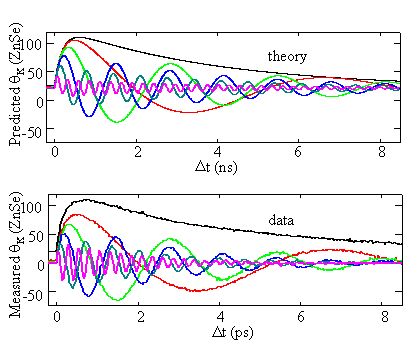Awschalom Group
Spontaneous Spin Transfer

Long electron spin lifetimes have been obtained in n-doped semiconductors, and coherent spin transport has been achieved over macroscopic distances in n-GaAs. In order to achieve spin dependent integrated electronics, it is necessary for spins to cross semiconductor interfaces without loss of quantum coherence. Based on our previous results (see electrons in semiconductors) we designed an experiment to test how spins cross interfaces between semiconductors with different band gaps, g-factors and doping concentrations:The coherent spin transfer can be measured using a variation of the Time Resolved Faraday Rotation technique in in reflection (Kerr) using different lasers to pump and probe. Since the band gap of GaAs is nearly half that of the ZnSe epilayer, it is possible to tune the pump to excite spin polarization only in the GaAs and probe only in the ZnSe:As the energy of the pump is tuned from below to above the absorption threshold of GaAs, coherent spin signal starts accumulating in the adjacent ZnSe epilayer (B=0):Unlike the case when spins are pumped and probed in the same layer, the rise on the spin signal is not instant but it takes hundreds of picoseconds. From the data shown above, a characteristic spin accumulation time can be extracted and it is found to be 200-400 ps (depending o the substrate doping).

When a magnetic field B is applied in the Voigt geometry, spins start precessing about the applied magnetic field at the Larmor frequency. We therefore probe an oscillating spin signal in the ZnSe epilayer where the frequency is proportional to the nominal gZnSe and to B:From the previous figure we see that as B is increased, the maximum spin signal decreases. Also, as illustrated below a phase shift appears.By taking into account the difference in g-factors between GaAs and ZnSe and the distribution of spin arrival times.we see that two spins excited at the same time in the GaAs but crossing the interface at different times will end-up "dephased" with respect to each other. This results in a (field dependent) decrease of the total spin amplitude and a non-zero phase. Using the fits to the B=0 data and taking into account the added dephasing at the interface, predictions can be made on how the spin signal evolves as the magnetic field is increased: